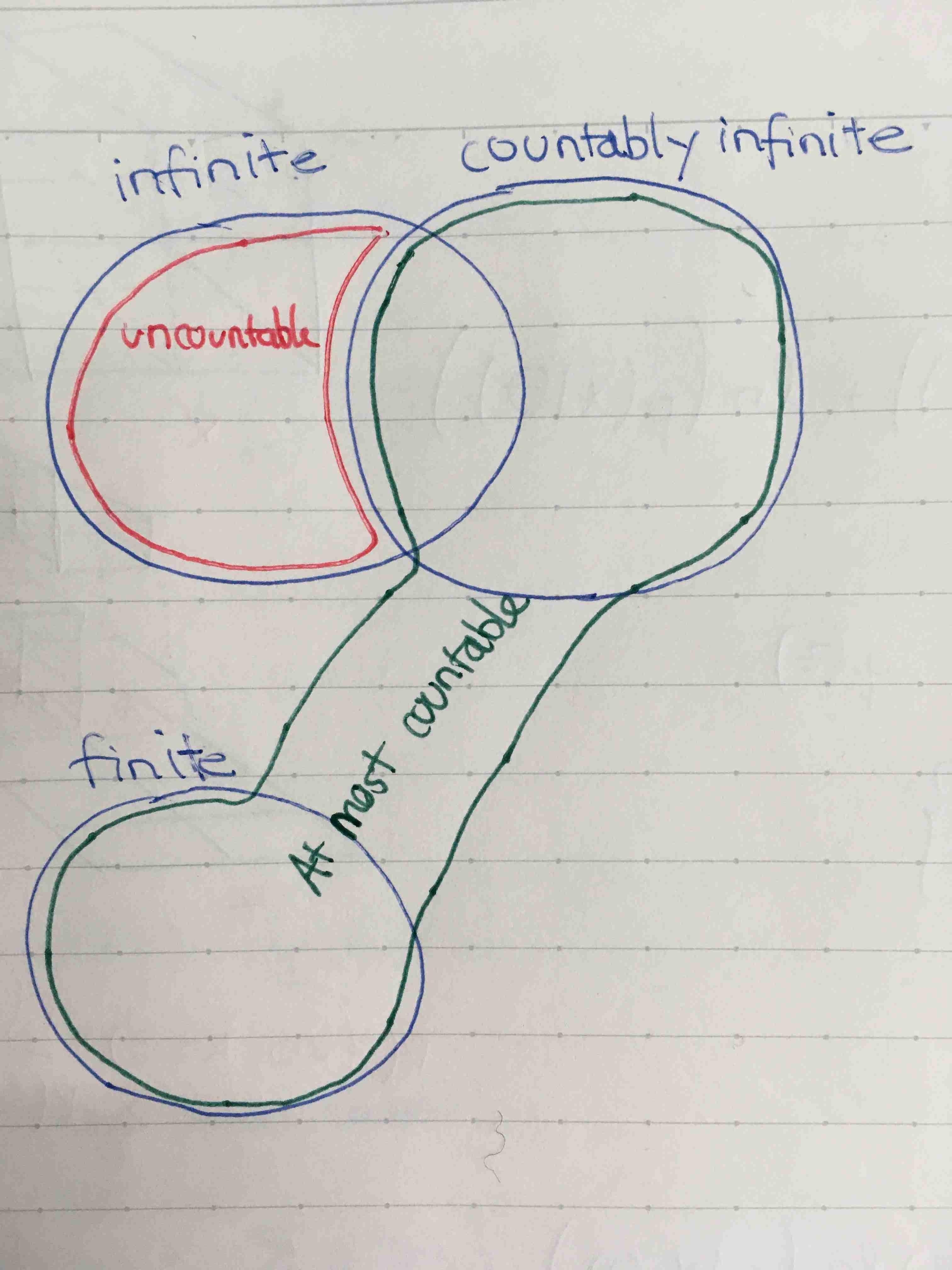Show Question
Math and science::Analysis::Tao::08. Infinite sets

# Countable sets

A set $$X$$ is said to be countably infinite (or just countable) iff it has equal cardinality with with the natural numbers $$\mathbb{N}$$

We also have the terms: at most countable and uncountable.

• A set is at most countable if it is either finite or coutable.
• A set is uncountable if it is infinite and not countable.
The relationship is shown below:Countably infinite sets are also called denumerable sets.

Note that a countable set must be infinite as it has the same cardinality as $$\mathbb{N}$$.

To understand the nature of countable sets, it is crucial to understand the nature of the bijection and how it operates on the infinite set $$\mathbb{N}$$. Recap below:

#### One-to-one functions

A function $$f$$ is one-to-one (or injective) if different elements map to different elements:

$x \neq x' \implies f(x) \neq f(x')$

#### Onto functions

A function $$f$$, $$f : X \rightarrow Y$$ is onto (or surjective) if every element in $$Y$$ can be obtained from applying $$f$$ to some element in $$X$$:

$\text{For every } y \in Y \text{, there exists an } x \in X \text{ such that } f(x) = y$

#### Bijective functions

A function which is both injective and surjective is called bijective or invertable.

### Example

We know that if $$X$$ is finite and $$Y$$ is a propper subset of $$X$$, then $$Y$$ does not have the same cardinality as $$X$$. This does not apply to infinite sets. For example, $$\mathbb{N}$$ has the same cardinality as $$\mathbb{N} - \{0\}$$, and thus, the latter is countable. This is so as we can have a bijection $$f: \mathbb{N} \rightarrow \mathbb{N} - \{x\}$$ from $$\mathbb{N}$$ to $$\mathbb{N} - \{x\}$$. (why? Look at the bijective definition above)

The set of even natural numbers, $$\{2n : n \in \mathbb{N} \}$$, is also countable.